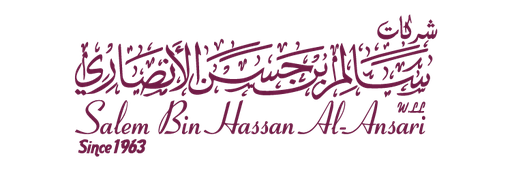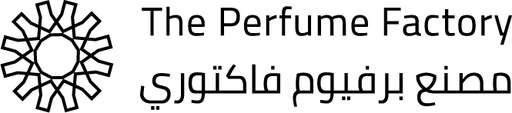# CAT - Technician

## Three views of the same cube are shown below. Which symbol is on the opposite side of X?*## In below figure, which of the cubes shown could be made from the pattern?*## In below figure, which of the patterns when folded will make the cube shown?*## In below figure, which symbol in the “Answer Figure” completes the sequence in the Problem Figure?*## In below figure, which of the Answer Figures fits the missing space in the Question Figure?*